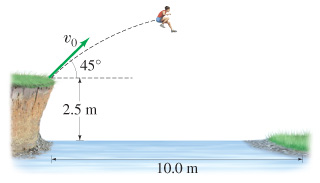# Problem: A long jumper leaves the ground at 45 above the horizontal and lands 9.1m away. A. What is her "takeoff" speed v0 ?B. Now she is out on a hike and comes to the left bank of a river. There is no bridge and the right bank is 10.0 m away horizontally and 2.5 m, vertically below. If she long jumps from the edge of the left bank at 45 with the speed calculated in A, how long, or short, of the opposite bank will she land? C. Will she land on the opposite bank?

###### FREE Expert Solution

In this problem, we're going to use the kinematic expression for the range to get the take-off speed.

$\overline{){\mathbf{R}}{\mathbf{=}}\frac{{{\mathbf{v}}_{\mathbf{0}}}^{\mathbf{2}}\mathbf{s}\mathbf{i}\mathbf{n}\mathbf{2}\mathbf{\theta }}{\mathbf{g}}}$, where R is the range and g is the acceleration due to gravity.

We'll also use the kinematic equation:

$\overline{){\mathbf{∆}}{\mathbf{y}}{\mathbf{=}}{\mathbf{v}}{\mathbf{t}}{\mathbf{-}}\frac{\mathbf{1}}{\mathbf{2}}{\mathbf{g}}{{\mathbf{t}}}^{{\mathbf{2}}}}$

91% (420 ratings)###### Problem Details

A long jumper leaves the ground at 45 above the horizontal and lands 9.1m away.A. What is her "takeoff" speed v0 ?

B. Now she is out on a hike and comes to the left bank of a river. There is no bridge and the right bank is 10.0 m away horizontally and 2.5 m, vertically below. If she long jumps from the edge of the left bank at 45 with the speed calculated in A, how long, or short, of the opposite bank will she land?

C. Will she land on the opposite bank?